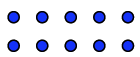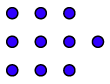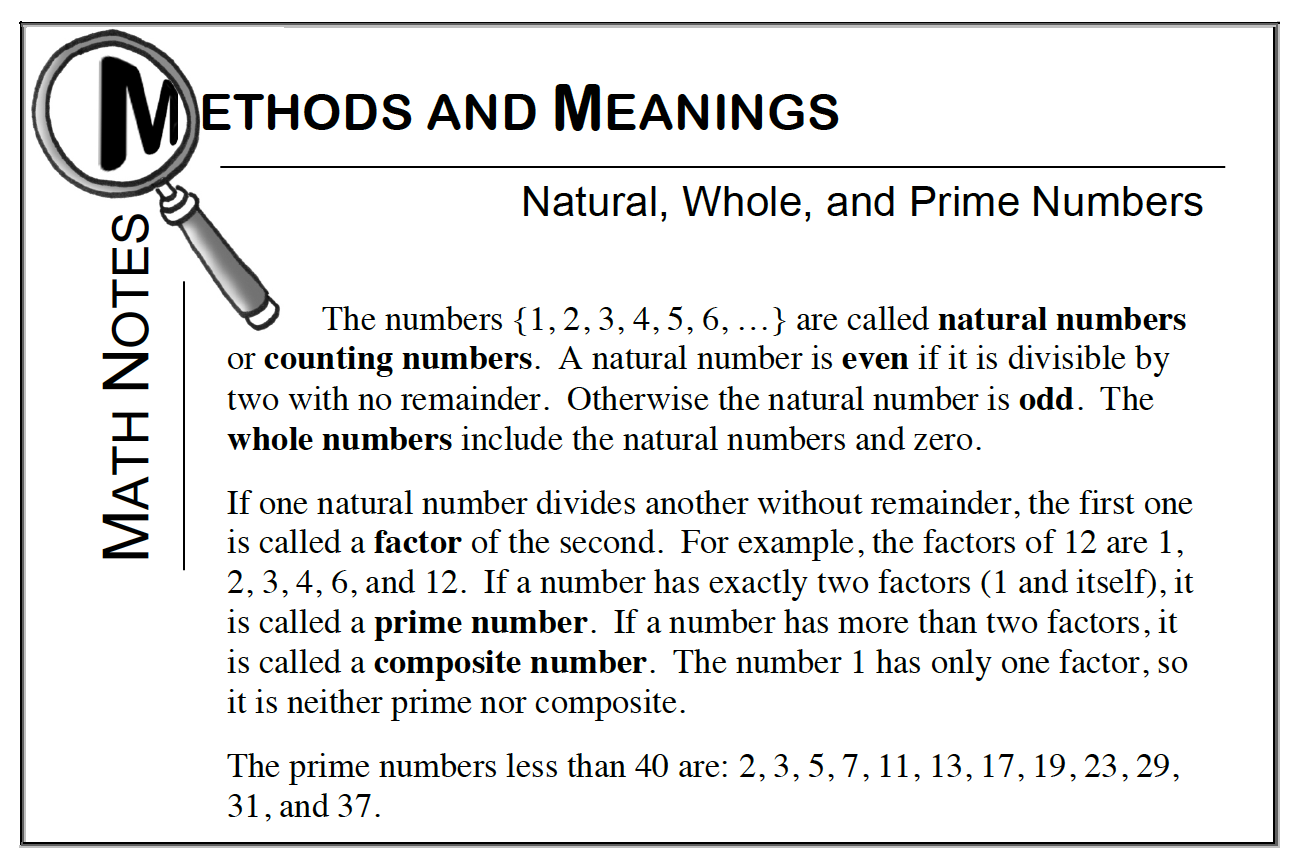### Home > CC1 > Chapter 1 > Lesson 1.2.3 > Problem1-69

1-69.

For each number of pennies below, arrange them first into a complete rectangular array and then into a different rectangular array that has a remainder of one (so there is one extra penny). Write an expression for each arrangement. Homework Help ✎

1. $10$ pennies

Try dividing $10$ by one or two to get your rectangle.To have a remainder of one, you want your rectangular array to be one less than $10$. That means you need to use the factors of 9 to get a rectangle for it.2. $15$ pennies

This one is very similar to the previous problem. If you are struggling with factors, look at the Math Notes box below.1. $25$ pennies

This problem is very similar to the previous two.